### Gail-Simon Test for Qualitative Interactions

The GAILSIMON option in the TABLES statement provides the Gail-Simon test for qualitative interaction for stratified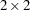tables. See Gail and Simon (1985), Silvapulle (2001), and Dimitrienko et al. (2005) for details.

The Gail-Simon test is based on the risk differences in stratifiedtables, where the risk difference is defined as the row 1 risk (proportion in column 1) minus the row 2 risk. See the section Risks and Risk Differences for details. By default, the procedure uses column 1 risks to compute the Gail-Simon test. If you specify the GAILSIMON(COLUMN=2) option, the procedure uses column 2 risks.

PROC FREQ computes the Gail-Simon test statistics as described in Gail and Simon (1985),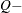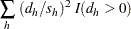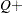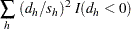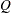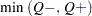where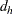is the risk difference in table,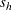is the standard error of the risk difference, and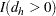equals 1 if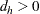and 0 otherwise. Similarly,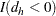equals 1 if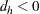and 0 otherwise. The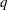tables (strata) are indexed by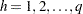.

The-values for the Gail-Simon statistics are computed as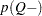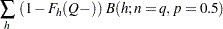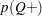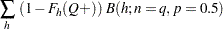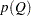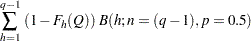where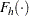is the cumulative chi-square distribution function withdegrees of freedom and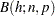is the binomial probability function with parametersand. The statistictests the null hypothesis of no qualitative interaction. The statistictests the null hypothesis of positive risk differences. A small-value forindicates negative differences; similarly, a small-value forindicates positive risk differences.